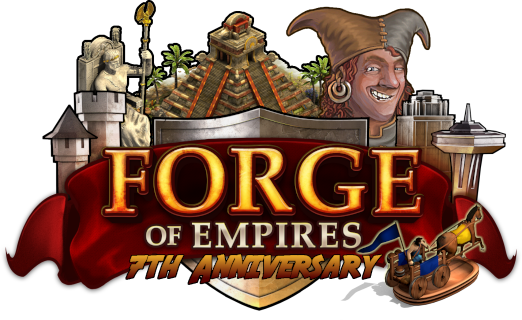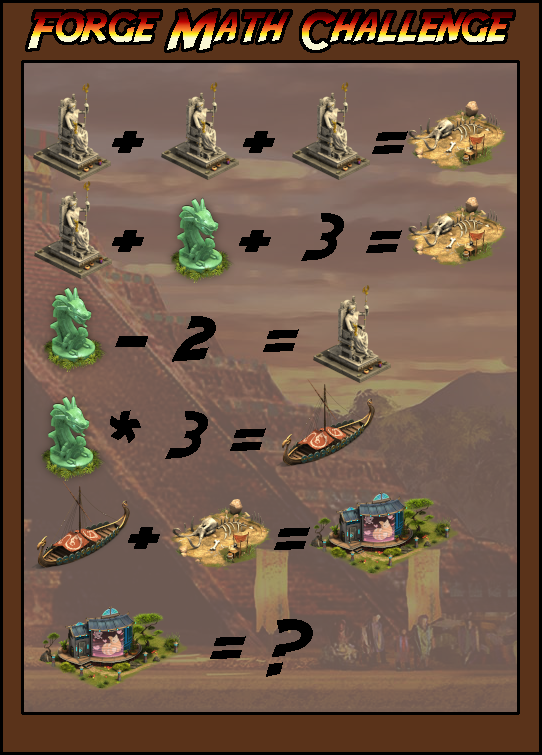# Contest day 6

Status
Not open for further replies.

36

36

#### DeletedUser40099

(zeus =5 bones= 15 dragon =7 boat= 21 stage=36) making the answer 36

54

#### DeletedUser39189

The ? = 36 (dino dig=15, longboat=21, because Zeus=5, Jade=7).

It is 36

#### DeletedUser39799

boat+skeleton pile

36

A=5
B=15
C=7
D=21
E=36

36

5+5+5=15
5+7=3=15
7-2=5
7*3=21
21+15=36
Building=36

Hibernus Mortis
East Nagash
USA server

36

#### DeletedUser39437Greetings, Queens and Kings!

2018 brought the Reconstruction Mode to the game, which was one of the most requested features of the game. The research "Mathematics" was needed to unlock it, but now it is on you to use some mathematics to solve the following puzzle!For this contest, 7 players can win 250 goods of your choice! Have fun calculating!

Please submit the correct answer until April 28, 2019. We will pick the 7 lucky winners from all correct answers!If you wish to leave any feedback about the Anniversary Contest, please click here.

Sincerely,
Your Forge of Empires Team

36

#### DeletedUser35502

Z=Zeus
D=Dragon
B=Bones
V=Viking ship
S=Stage

D-2=Z //+2
D = Z + 2

3Z = Z + D + 3
3Z = Z + (Z+2) + 3
3Z = 2Z + 5 //-2Z
Z = 5

3Z= B = 15
Z + D + 3 = 15
5 + D + 3 = 15 //-8
D = 7

D * 3 = V
7 * 3 = V = 21
V + B = S
21 + 15 = S = 36

Last edited by a moderator:

#### DeletedUser39866

The answer is 36.

36

#### DeletedUser36335

The house is equal to 36.
Statue of Zeus=5
Incident=15
Relic=7
Langship=21

Last edited by a moderator:

26

sorry 36

#### DeletedUser37938

36!

Status
Not open for further replies.